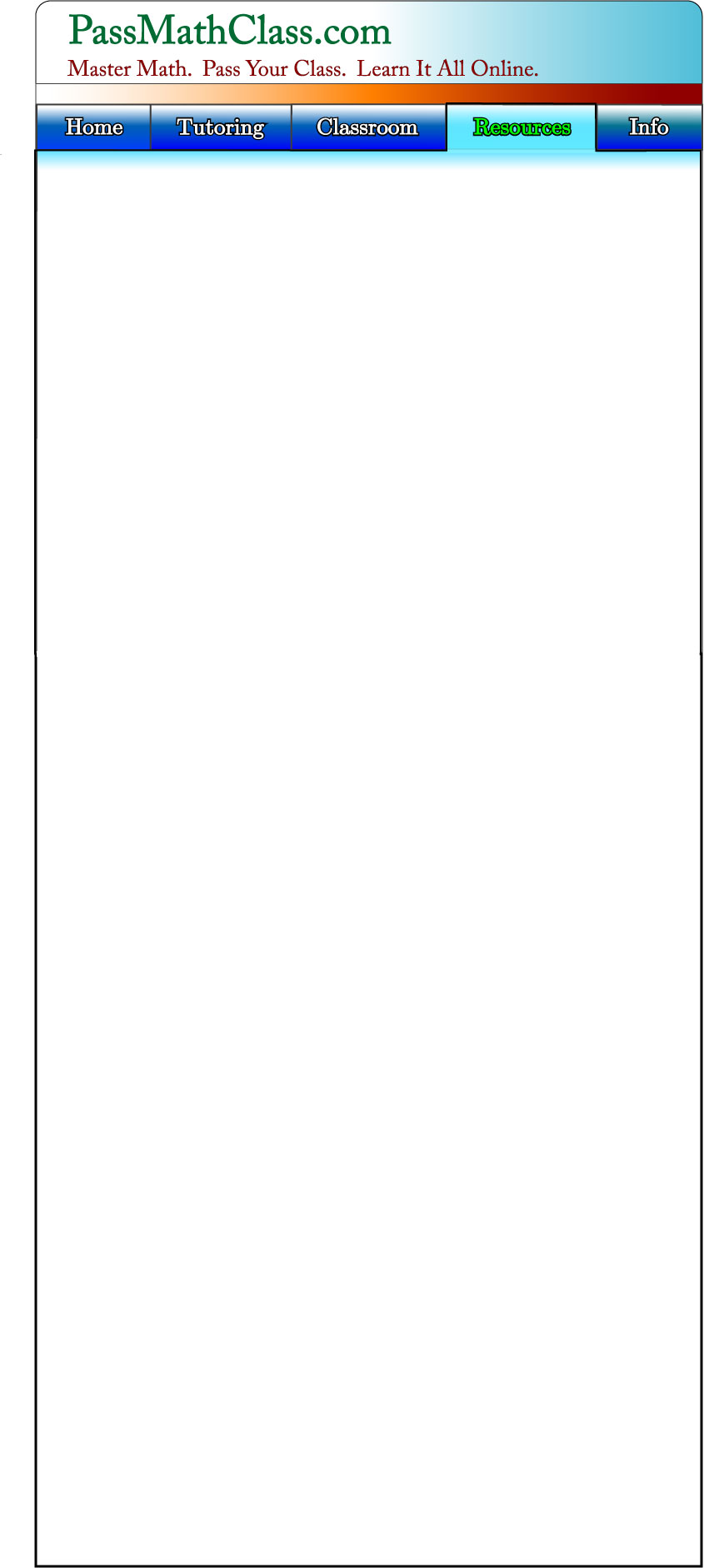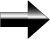The Properties of Real Numbers

All numbers follow basic rules for how they can be manipulated.  It is extremely important for you to understand these rules if you want to master math (or even just pass it).  All the rest of algebra is based on these rules.  The good news is that you are probably very familiar with most or all of them.  Here are the rules for Real Numbers:

In these definitions, let a, b, and c be real numbers.  This type of general definition is very common in math.  Rules are written out in variables instead of actual numbers.  It is also important for you to understand how to read and interpret mathematical definitions.

Look through these rules and make sure that you completely understand the concepts behind each of them.

The Rules

Commutative Properties:

a + b = b + a -- It doesn’t matter if you add 1 to 2 or 2 to 1, both are equal to 3.

a * b = b * a -- The order doesn’t matter when you multiply, either.  2*3 = 3*2.

Associative Properties:

(a + b) + c = a + (b + c) -- Again, since these are additions and multiplications, the order doesn’t matter.  Here we have just re-grouped the terms using parentheses.

(a * b) * c = a * (b * c) -- For Example, (1 * 2) * 3 = 1 * (2 * 3) = 6

Identities:

a + 0 = a -- These seem obvious, but they are actually rules.  If you add zero to something, its value doesn't change.

a * 1 = a -- Another obvious rule: Any number times one is itself. An identity is like an obvious fact, truly basic.

Inverses:

These are essentially definitions of subtraction and division.  If you add the opposite of a number to itself, you get zero.  It’s like digging a hole: the sand is the positive and the hole is the negative. If you put the sand back in the hole, you get level ground --- zero.

a + (-a) = 0

a * (1 / a) = 1, and a ≠ equal 0 (for the reason for this, click here).

Distributive Property:

a(b + c) = ab + ac

Subtraction and Division:

a – b = a + (-b)

a / b = a * (1/b)

It’s very important for you to understand all of these concepts and rules. The rest of algebra is based on them. If you develop a solid foundation, you will be able to truly master all the rest of algebra.  Math is all about building on the knowledge you already have.

Main Pre-Algebra Page